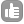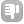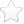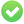1Is there a formula that would suggest a reasonable starting value for the mixing time based on the molecular weight, magnet field strength and solvent viscosity? Would anyone recommend some publication on the subject? Thanks! asked Aug 16 '09 at 14:47Evgeny Fadeev 577●14A mixing time that is about 1/3 to 1/2 of T1 will give maximum NOE. To estimate distances you need to be in the linear region of the transfer, or fit an entire mixing series. To get data in the linear region us a mixing time that is about 5% of T1. Since there is no hard relationsip betwen T1 and Mw or viscosity there is no equation such as the one you are asking for. There may be approximations which are true within a limited family of polymers, but there is no general realationship. answered Aug 18 '09 at 12:29dare 61●14There IS a relationship between `T1` and correlation time which in turn is dependent on `MW` and viscosity. You may obtain a rough estimate of `T1` using the following relationships. The relationship between rotational correlation time and `T1` is given by the Bloembergen, Purcell and Pound equation (BPP equation) see (relaxation time section in `http://en.citizendium.org/wiki/Nmr_spectroscopy#NMR_spectroscopy:_Observable_parameters` ). The correlation time can be estimated from knowledge of MW and viscosity as follows: Assuming a spherical shape, the radius of hydration `rh = ((MW)^0.44)/1.5` Use this radius, `rh`, to calculate volume of hydration, `Vh`. According to the Stokes-Einstein relation, the rotational diffusion coefficient `Dr = k*T/(6*Vh*viscosity)` And Rotational correlation time `tau_r = 1/(6*Dr)` Therefore, rotational correlation `tau_r = Vh*viscosity/(k*T)` `k=Boltzmann` constant and `T=Temperature` References: Cantor and Schimmel : Biophysical Chemistry M.Goldman : Quantum description of high-resolution NMR in liquids. answered Feb 11 '10 at 21:28Evgeny Fadeev 577●1 Dr. Talluri, I've added little fixes to formatting so that equations show better. - Evgeny Fadeev (Feb 12 '10 at 12:33)

 toggle preview

Tags:

×26
×11

Asked: Aug 16 '09 at 14:47

Seen: 8,195 times

Last updated: Feb 12 '10 at 12:32